# LTE Metrics including RSRP, RSRQ and SINR

Summary:

RSRQ and SINR are key metrics for LTE systems including CableFree LTE-A Infrastructure.  This page explains the technical differences.

In LTE – as any wireless system – the performance has a direct relationship with the RF conditions at the time.  To aid with performance analysis in LTE, it is typical to define some ranges of RF measurements that correspond to some typical RF conditions one might find themselves in.

The table below represents a good classification of RF conditions Vs LTE KPIs.  The source of this table is a vendor and has been complied during the RF tuning process in the field for an operator. Of course there are no rules as to how various RF conditions are classified, so different tables will exist but to a great extent you can expect them to align.

## LTE Metrics including RSRP, RSRQ and SINR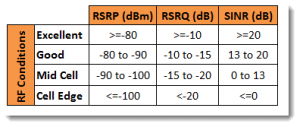In this particular example, three measurement quantities are used

• RSRP (Reference Signal Received Power)
• RSRQ (Reference Signal Received Quality)
• SINR (Signal to Interference & Noise Ratio)

You can find more info about RSRP & RSRQ in our page: RSRP and RSRQ Measurement in LTE

## LTE SINR Definition

SINR is defined as:

 S: indicates the power of measured usable signals. Reference signals (RS) and physical downlink shared channels (PDSCHs) are mainly involved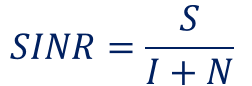I: indicates the average interference power – the power of measured signals or channel interference signals from other cells in the current system N: indicates background noise, which is related to measurement bandwidths and receiver noise coefficients

All quantities are measured over the same bandwidth and normalized to one subcarrier bandwidth.

SINR is a measure of signal quality as well but it is not defined in the 3GPP specs but defined by the UE vendor.

It is not reported to the network. SINR is used a lot by operators, and the LTE industry in general, as it better quantifies the relationship between RF conditions and Throughput. LTE UEs typically use SINR to calculate the CQI (Channel Quality Indicator) they report to the network.

It is a common practice to use Signal-to-Interference Ratio (SINR) as an indicator for network quality. It should be however noted that 3GPP specifications do not define SINR and  therefore UE does not report SINR to the network. SINR is still internally measured by most UEs and recorded by drive test tools.

Unfortunately UE chipset and RF scanner manufacturers have implemented SINR measurement in various different ways which are not always easily comparable. While at first it may seem that defining SINR should be unambiguous, in case of LTE downlink this is not the case. This is because different REs within a radio frame carry different physical signals and channels each of which, in turn, see different interference power depending on inter-cell radio frame synchronization.

For example, in a frame-synchronized network, SINR estimation based on synchronization signals (PSS/SSS) results in different SINR than SINR estimation based on Reference Signals, since in the latter case the frequency shift of the RS depends on the PCI plan.

## LTE RSRQ Definition

RSRQ is defined as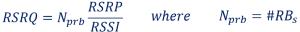In what follows we show one way of converting RSRQ to SINR.

In OFDM own cell interference is often assumed to be negligible and consequently I is due to other cell interference only.

RSSI is defined as: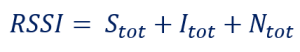where the subscript ’tot’ indicates that the power is measured over the 12NRE subcarriers of the measurement bandwidth.

The total serving cell received power depends on the number of transmitted subcarriers in the OFDM symbol carrying R0, and on the number of transmit antennas.

We can model this impact using the per-antenna subcarrier activity factor x and set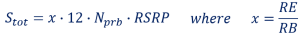The value of x = 1 indicates full load such that all subcarriers of one transmit antenna are transmitted for the OFDM symbol carrying R0

If only RS is transmitted (i.e., unloaded cell) the resulting subcarrier activity factors would be x = 1/6 and x = 1/3 for one and two transmit antennas, respectively.

When calculating x for two transmit antennas, one should take into account that REs overlapping with adjacent antenna RS transmission are muted, and therefore, for example, in a fully loaded 2Tx cell the scaling factor is x = 5/3 , instead of two.

It is assumed that all subcarriers have the same power, i.e., there is no power boosting for any channel.

Since subcarrier interference plus noise power isby combining the above equations we have the following relation of average subcarrier SINR and RSRQ (Click on Formula To Enlarge):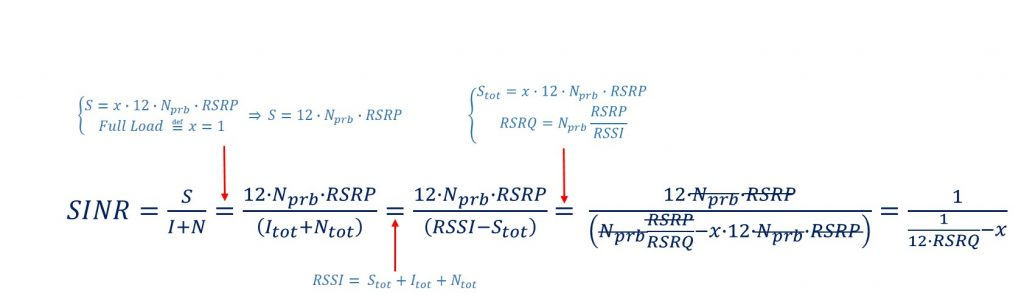and finally: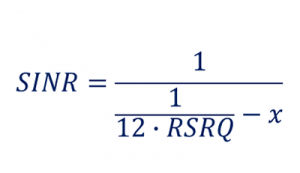## Graph showing SINR vs RSRQ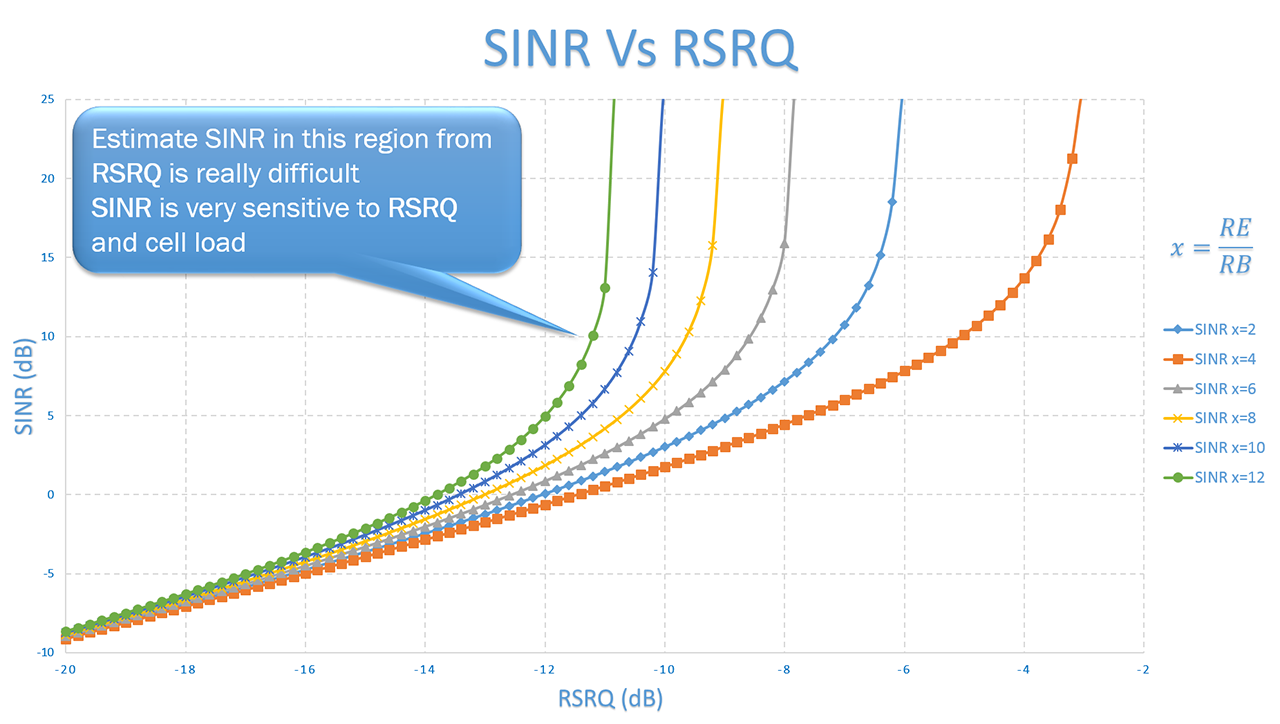One uncomfortable property of the RSRQ to SINR mapping is that it depends on the instantaneous serving cell subcarrier activity factor x, which is typically not known in live network measurements.

The value of x = 1 indicates full load such that all subcarriers of one transmit antenna are transmitted for the OFDM symbol carrying R0.

If only RS is transmitted (i.e., unloaded cell) the resulting subcarrier activity factors would be x = 1/6 and x = 1/3 for one and two transmit antennas, respectively

#### When calculating x for two transmit antennas, one should take into account that REs overlapping with adjacent antenna RS transmission are muted, and therefore, for example, in a fully loaded 2Tx cell the scaling factor is x = 5/3 , instead of two

Another problem is the sensitivity of the mapping on RSRQ values; a small change in RSRQ can result in a very large change in SINR which makes such mapping difficult to use in fading radio conditions.

Indeed, plotting a RSRQ versus SINR scatter graph from a drive test measurement one rarely obtains such a nicelooking curve as shown in the Figure above.

Currently available measurement UEs and scanners report SINR directly.